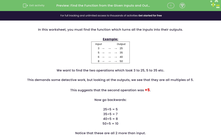# Find Functions or Numbers in Input/Output Tables

In this worksheet, students will fill in missing functions or numbers in input/output tablesKey stage:  KS 3

Curriculum topic:   Algebra

Curriculum subtopic:   Translate Situations/Procedures into Expressions, Formulae and Graphs

Popular topics:   Algebra worksheets, Year 7 Algebra worksheets

Difficulty level:#### Worksheet Overview

In this activity, we will be finding the function that turns all the inputs into their outputs.

Example:

Input   Output
9   3
27   9
6   2
15   5

In the example above, we have to work out what should go in the middle that turned the input into the output.

If we look at the two columns, we can see that there is a pattern. Each of the numbers in the input column has been divided by 3 to get the answer in the output column. So, each of those empty boxes needs to have ÷ 3 written in.

If x is the missing function, then we can write this as x ÷ 3 or x/3

Sometimes, we will have two steps to follow to find the function.

Input   Output
3 25
5 35
6 40
8 50

We want to find the two operations that took 3 to 25, 5 to 35 etc.

This demands some detective work, but looking at the outputs, we see that they are all multiples of 5.

This suggests that the second operation was x 5.

Now go backwards:

25 ÷ 5 = 5
35 ÷ 5 = 7
40 ÷ 5 = 8
50 ÷ 5 = 10

Notice that these are all two more than the input. So, the first empty boxes should be + 2

If we call the function x, then we get  (x + 2) x 5

This might look a bit tricky, but the key is to look carefully at the numbers in the output column and see if there is anything that they have in common.

Are you ready to have a go?### What is EdPlace?

We're your National Curriculum aligned online education content provider helping each child succeed in English, maths and science from year 1 to GCSE. With an EdPlace account you’ll be able to track and measure progress, helping each child achieve their best. We build confidence and attainment by personalising each child’s learning at a level that suits them.

Get started#### Popular Maths topics

••••